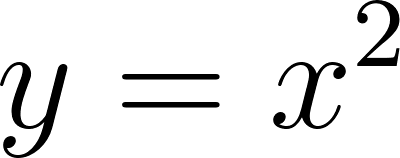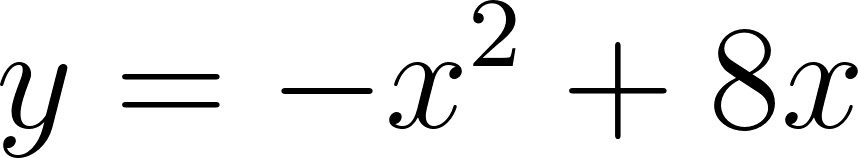×
Get Full Access to Calculus: Early Transcendentals - 1 Edition - Chapter 1.2 - Problem 41e
Get Full Access to Calculus: Early Transcendentals - 1 Edition - Chapter 1.2 - Problem 41e

×

# Solved: Intersection problems Use analytical methods toISBN: 9780321570567 2

## Solution for problem 41E Chapter 1.2

Calculus: Early Transcendentals | 1st Edition

• Textbook Solutions
• 2901 Step-by-step solutions solved by professors and subject experts
• Get 24/7 help from StudySoup virtual teaching assistantsCalculus: Early Transcendentals | 1st Edition

4 5 1 363 Reviews
10
1
Problem 41E

Intersection problems:Use analytical methods to find the following points of intersection. Use a graphing utility only to check your work. Find the point(s) of intersection between the parabolasandStep-by-Step Solution:

Step 1 of 4

Given,

The equation of Parabola one,The equation of Parabola two,Step 2 of 4

Step 3 of 4

##### ISBN: 9780321570567

Unlock Textbook Solution